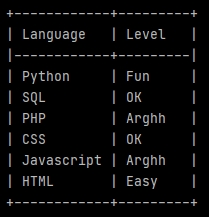### Merge/concatenate

#### Merge 2 lists in 2 columns and properly display them in the terminal```import pandas as pd
from tabulate import tabulate

LanguageList = ['Python', 'SQL', 'PHP', 'CSS', 'Javascript', 'HTML']
LevelList = ['Fun', 'OK', 'Arghh', 'OK', 'Arghh', 'Easy']

LanguageLevel = list(zip(LanguageList, LevelList))

df = pd.DataFrame(LanguageLevel, columns =['Language', 'Level'])

print(tabulate(df, headers='keys', tablefmt='psql', showindex=False))```

The 7th line uses `zip` to link the values from our lists in tuples`list` encloses our tuples in a list.

The 9th line creates a data-frame (`pd.DataFrame`) from our new list (now containing tuples). Specifying the column labels.

The print uses `tabulate` to display our fields with a beautiful Postgres style (`psql`).

#### Split a dataframe with conditions, and them merge the splits according other conditions

```firstSelection = df.loc[df['Field'] == 'Yes'].head(100)

secondSelection = df.loc[df['Field'] == 'No'].head(200)

# Merge the 2 selections.
df_Selections = pd.concat([firstSelection, secondSelection], axis=0)```

#### Concatenate dataframes created in a loop

```df_Concatenation = pd.DataFrame()

for ... :
df...
df_Concatenation = pd.concat([df_Concatenation, df], axis=0)```